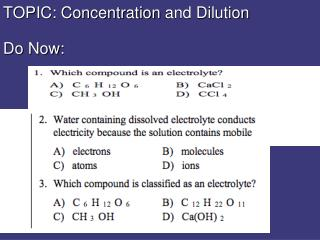Download PresentationTOPIC: Concentration and Dilution Do Now:

# TOPIC: Concentration and Dilution Do Now: - PowerPoint PPT PresentationDownload Presentation## TOPIC: Concentration and Dilution Do Now:

- - - - - - - - - - - - - - - - - - - - - - - - - - - E N D - - - - - - - - - - - - - - - - - - - - - - - - - - -
##### Presentation Transcript

1. TOPIC: Concentrationand DilutionDo Now:

2. Parts of a Solution • Solute= dissolved substance • Solvent= dispersing medium

3. We can determine how much solute and solvent make up a solution = CONCENTRATION • Concentration = A measurement that describes how much solute is in a given amount of solvent or solution. • Many, many different units to express concentration. • Molarity • PPM (parts per million) • % by mass • % by volume

4. Molarity (M) Molarity (M) = moles of solute liters of solution CAN USE THIS TO HELP CALCULATE moles M liters

5. So why do aqueous solutions need .1M or 3M in front?!?!?!

6. VS

7. Dilution-which one has a higher molarity?

8. Amount of solute is constantAmount of solvent increases Dilution = add water = less concentrated Amount solute in concentrated solution EQUALS Amount solute in dilute solution

9. MC x VC = MD x VD (C = concentrated D = dilute) MolarityC x VolumeC = MolarityD x VolumeD

10. 300ml of 2.5M NaOH is diluted to 750ml. Find the molarity of the diluted solution MC x VC = MD x VD 300ml x 2.5M = 750ml x MD So how much water did we have to add to delete the 2.5 M solution? water ?M 750 -300 _ 450ml 1M 2.5M 2.5M 300ml 750ml

11. Example: We are doing a lab and the procedure calls for 300ml 1.2 M NaOH. However, all we have is a bottle of 5.5M NaOH. We have to dilute it!!!! The Equation: MC x VC = MD x VD 5.5 M x VC = 1.2 M x 300ml V1 = (1.2 M x 300 ml) 5.5 M V1 = 0.065 L = 65 mL So what does 65 ml tell us?!

12. To prepare the 1.2 M NaOH solution, you pour 65 mL of 5.5 M NaOH into your container and add water to get 300 mL final volume (300-65 = 235 ml water added)